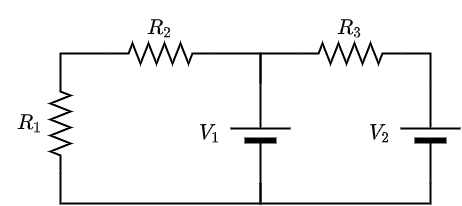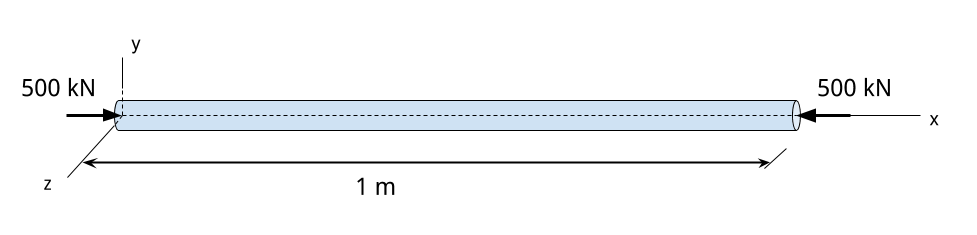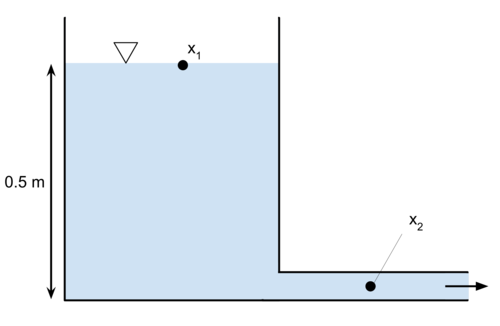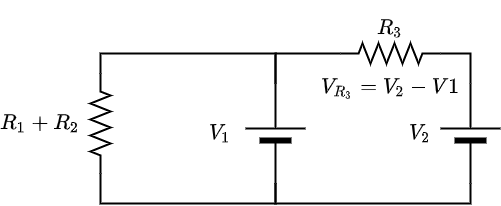Free FE Practice Test

# PrepFE™

## Free FE Mechanical Example Practice Problems

We've selected 10 diverse practice problems from our question bank that you can use to review for the Mechanical engineering FE exam and give you an idea about some of the content we provide.

### 1) Given the values below, what is nearest the total power dissipated for this circuit? $R_1 = \SI{10}{\Omega}$, $R_2 = \SI{3}{\Omega}$, $R_3 = \SI{8}{\Omega}$ $V_1 = \SI{5}{V}$, $V_2 = \SI{8}{V}$### 4) The plot below represents the movement of a block on a rough surface due to an external force. Select all that represent the line segment $b$.### 5) An aluminum rod with diameter of $d = \SI{12}{\milli\meter}$ is loaded axially in compression as shown. Using a Young's modulus of $\SI{70}{\giga\pascal}$ and Poisson ratio of 0.35, what is most nearly the increase in diameter of the rod at the midspan of the rod? Assume the internal loading at the midspan of the rod is -500 kN.### 6) The water velocity at $x_2$ as the water exits the system into the atmosphere is most nearly:### 9) In the stress-strain diagram, select all that point A describes.## Solutions

### 1) Given the values below, what is nearest the total power dissipated for this circuit? $R_1 = \SI{10}{\Omega}$, $R_2 = \SI{3}{\Omega}$, $R_3 = \SI{8}{\Omega}$ $V_1 = \SI{5}{V}$, $V_2 = \SI{8}{V}$A.$1.1 \si W$
B.$3.0 \si W$
C.$7.1 \si W$
D.$21 \si W$

### Explanation:

Refer to the Power Absorbed by a Resistive Element section in the Electrical and Computer Engineering chapter of the FE Reference Handbook.

Use the equation, $$P = \frac{V^2}{R}$$ The total power dissipated in the circuit is the sum of the powers consumed by each individual resistor. Write an expression for the power consumed on each individual resistor and sum them together, $$P_{\text{total}} = \frac{(V_2-V_1)^2}{R_3} + \frac{(V_1)^2}{R_1+R_2}$$ $$= \frac{(\SI{8}{V}-\SI{5}{V})^2}{\SI{8}{\Omega}} + \frac{(\SI{5}{V})^2}{\SI{13}{\Omega}}$$ $$\approx \SI{3.05}{W}$$ Remember that the $V$ in the equation $\frac{V^2}{R}$ refers to the voltage drop across the resistor. For the resistor, $R_3$, this is the difference between $V_2$ and $V_1$.

For the resistors $R_1$ and $R_2$, their total power consumption can be found by adding them together and dividing them into the voltage drop across both of them, which is simply the voltage $V_1$.

Another way to look at this is that the resistors $R_1$ and $R_2$ are together in series which are then in parallel with the voltage $V_1$. The voltage drop across the series combination of resistors is $V_1 - 0 = V_1$ because all the voltage sources share a common reference. Thus, the term $V_1$ is all that ends up in that particular term of the total power equation.### 2) The following three lines form a triangle with vertices at what points? \begin{align} x+3y=1 \\ 2x+y=0 \\ -2x+y=0 \end{align}

A.$(- \frac{1}{5},\frac{2}{5}), (0,0), (\frac{1}{7}, \frac{2}{7})$
B.$(- \frac{1}{5},\frac{2}{5}), (1,1), (\frac{1}{7}, \frac{2}{7})$
C.$(- \frac{1}{5},-\frac{2}{5}), (0,1), (\frac{1}{7}, \frac{2}{7})$
D.$(\frac{1}{5},\frac{2}{5}), (0,0), (\frac{1}{7}, \frac{2}{7})$

### Explanation:

Find the intersection for each pair of lines. Using the initial two equations, let's try substituting the second into the first by solving the second for y: \begin{align} y &= -2x \\ \Rightarrow x+3(-2x)&=1 \\ -5x &= 1 \\ x &= - \frac{1}{5} \end{align} Then plug that back into either of the first two equations to find the y-coordinate of the intersection. $$y = -2(- \frac{1}{5}) = \frac{2}{5} \\ \rightarrow (x,y)=(- \frac{1}{5},\frac{2}{5})$$ Now, we do the same thing twice for the second and third equations and then the first and third equations. For the second and third equations, we see that $y=-y$, so the second intersection we know that only (0,0) will work. For the last pair: \begin{align} y &= 2x \\ \Rightarrow x+3(2x)&=1 \\ 7x &= 1 \\ x &= \frac{1}{7} \\ \rightarrow (x,y) &= (\frac{1}{7}, \frac{2}{7}) \end{align} We have: $$(- \frac{1}{5},\frac{2}{5}), (0,0), (\frac{1}{7}, \frac{2}{7})$$

A.34 m/s
B.97 m/s
C.60 m/s
D.50 m/s

### Explanation:

Refer to the Particle Rectilinear Motion section in the Dynamics chapter of the FE Reference Handbook. $$a = \mathrm{d}v/\mathrm{d}t$$ $$v = \int a\,\mathrm{d}t$$ $$v = \frac{2}{3}t^3 + 2t + v_0$$ $$= \frac{2}{3}(3)^3 + 2(3) + 10$$ $$= \SI{34}{m/s}$$ Refer to the Indefinite Integrals section in the Mathematics chapter of the FE Reference Handbook to see a list of typical integration identities. Alternatively, you could solve this integral straight from the calculator using the integration feature in your TI-36X Pro calculator.

### 4) The plot below represents the movement of a block on a rough surface due to an external force. Select all that represent the line segment $b$.The correct answers are A, B, and D.

### Explanation:

The block begins moving and remains in motion during $b$ because $x(t)$ is increasing. This is where the coefficient of kinetic friction applies. Work is being done because a force is acting over a distance.

Also, because there is motion but no acceleration on the block, it's also in dynamic equilibrium.

### 5) An aluminum rod with diameter of $d = \SI{12}{\milli\meter}$ is loaded axially in compression as shown. Using a Young's modulus of $\SI{70}{\giga\pascal}$ and Poisson ratio of 0.35, what is most nearly the increase in diameter of the rod at the midspan of the rod? Assume the internal loading at the midspan of the rod is -500 kN.A.$\SI{49}{\micro\meter}$
B.0.31 mm
C.$\SI{265}{\micro\meter}$
D.230 nm

### Explanation:

Refer to the Uniaxial Stress-Strain section in the Mechanics of Materials chapter of the FE Reference Handbook.

Ultimately, we must determine the increase in rod diameter after the rod is compressed. We can determine the increase in length of a member from its engineering strain. $$\epsilon=\Delta L/L_0$$ Which can be interpreted as $$\epsilon_{lateral}=\Delta L_{lateral}/L_{0 lateral}=\Delta d/d_0$$ Solve for the unknowns. Ultimately, we must solve for $\Delta d$.
$d_0=12\si{mm}=0.012\si{m} \\ \Delta d=? \\ \epsilon_{lateral}=?$

Side note: lateral strain refers to strain that occurs to the sides of the rod (cross section) and NOT strain that occurs along the length of the rod, that would be longitudinal strain. Therefore the equation above was set up in terms of lateral strain because this problem asks for the change in length laterally (at the cross section). Solve for lateral strain using Poisson's ratio. $$\nu=\frac{-\text{lateral strain}}{\text{longitudinal strain}}=0.35=\frac{-\text{lateral strain}}{\text{longitudinal strain}}$$ Solve for longitudinal strain using Hooke's law, so we can then come back and solve for lateral strain. $$E=\sigma/ \epsilon_{longitudinal}\rightarrow E=(P/A)/\epsilon_{longitudinal}$$ Solve for the unknowns.
$E= 70\times 10^9\si{Pa} \\ A=\pi/4\cdot d^2=\pi/4\cdot (0.012\si{m})^2=0.000113097 \si{m^2} \\ P=\text{internal loading}=500\si{kN}=500,000\si{N}$ $$70\times 10^9\si{Pa}=(-500,000\si{N}/0.000113097 \si{m^2})/\epsilon_{longitudinal} \\ \therefore \epsilon_{longitudinal}=-0.0631569$$ We can go back and solve for lateral strain using Poisson's ratio. $$0.35=\frac{-\text{lateral strain}}{\text{longitudinal strain}}=\frac{-\epsilon_{lateral}}{-0.0631569}$$ $$\therefore \epsilon_{lateral}=0.022104915$$ We can go back to the first equation and solve for the change in diameter. $$\epsilon_{lateral}=\Delta d/d_0 \\ 0.022104915=\Delta d/0.012\si{m};\text{solve for } \Delta d \\ \Delta d = 0.000265259\si{m}=\SI{265}{\micro\meter}$$ Some of the takeaways from this problem are:
Strain and deformation to a rod can occur longitudinally (along the length of the rod) or laterally (along the cross section diameter length). Therefore, you can use any of the Hooke's Law equations and modify them to be in terms of lateral deformation or longitudinal deformation. In this problem, the rod got shorter and the diameter got larger as the two 500 kN pushed into the rod axially.

### 6) The water velocity at $x_2$ as the water exits the system into the atmosphere is most nearly:A.3.1 m/s
B.6.1 m/s
C.7.0 m/s
D.11 m/s

### Explanation:

Refer to the Principles of One-Dimensional Fluid Flow section in the Fluid Mechanics chapter of the FE Reference Handbook.

Ultimately, we must solve for the velocity at the outlet of the system, $v_2$. Use Bernoulli's equation to solve for $v_2.$ $$\frac{p_2}{\gamma} +\frac{v_2^2} {2g} +z_2 = \frac{p_1}{\gamma} + \frac{v_1^2} {2g} +z_1$$ Calculate the unknowns:

$p_1=0 \si{Pa}$ because the water is exposed to the atmosphere, which is 0 gauge pressure or 101.3 kPa absolute pressure
$\gamma_{water}=9.81\si{kN/m^3}$
$z_1=0.5\si{m}$
$g=9.81\si{m/s^2}$
$v_1=0$ since the water at $x_1$ is just sitting there, not moving
$v_2=?$
$p_2=0 \si{Pa}$ because the water is released to the atmosphere, which is 0 gauge pressure or 101.3 kPa absolute pressure
$z_2=0$ $$\frac{0}{9.81\si{kN/m^3}} +\frac{v_2^2} {2(9.81\si{m/s^2})} +0 = \frac{0}{9.81\si{kN/m^3}} \\ + \frac{0^2} {2(9.81\si{m/s^2})} +0.5\si{m}; \text{solve for }v_2$$ $$v_2=3.1\si{m/s}$$

### 7) A parallel flow heat exchanger operates with water ($c_{p,w} = \SI{4.186}{\kilo\joule\per\kilogram\per\kelvin}$) and oil ($c_{p,o} = \SI{3.4}{\kilo\joule\per\kilogram\per\kelvin}$). Water, the cold fluid, enters the inner tube at $\SI{300}{\kelvin}$ and leaves at $\SI{400}{\kelvin}$. What is the log mean temperature difference if oil, the hot fluid, enters the heat exchanger at $\SI{550}{\kelvin}$ and leaves the system at $\SI{450}{\kelvin}$?

A.$\SI{240}{\kelvin}$
B.$\SI{234}{\kelvin}$
C.$\SI{108}{\kelvin}$
D.$\SI{124}{\kelvin}$

### Explanation:

According to the FE Reference Handbook, the log mean temperature difference for parallel flow heat exchangers is defined as $$\Delta T_{lm} = {(T_{Ho} - T_{Co}) - (T_{Hi} - T_{Ci}) \over ln \left ( T_{Ho} - T_{Co} \over T_{Hi} - T_{Ci} \right )}$$ where $T_{Hi}$ the hot fluid inlet temperature, $T_{Ho}$ the hot fluid outlet temperature, $T_{Ci}$ the cold fluid inlet temperature and $T_{Co}$ the cold fluid outlet temperature. In our problem water is the cold fluid and oil the hot one, therefore we have $$\Delta T_{lm} = {(T_{oil,out} - T_{water,out}) - (T_{oil,in} - T_{water,in}) \over ln \left ( T_{oil,out} - T_{water,out} \over T_{oil,in} - T_{water,in} \right )} \\ = {(\SI{450}{\kelvin} - \SI{400}{\kelvin}) - (\SI{550}{\kelvin} - \SI{300}{\kelvin}) \over ln \left (\SI{450}{\kelvin} - \SI{400}{\kelvin} \over \SI{550}{\kelvin} - \SI{300}{\kelvin} \right )} \\ = \SI{124.3}{\kelvin}$$

A.0.51
B.2.18
C.1.93
D.1.20

### Explanation:

Refer to the Dispersion, Mean, Median, and Mode Values section in the Engineering Probability and Statistics chapter of the FE Reference Handbook.

The population is 50, but the sample is 9. Since we only have pressure values for 9 measurements out of the 50 total measurements, we must use the sample standard deviation formula. Ultimately, we must solve for: $$s = \sqrt{ \frac{1}{(n-1)} \sum_{i=1}^n \left(X_i-\bar{X}\right)^2 }$$ Solve for the unknowns.
The total number of samples is $n=9$.
Calculate the arithmetic mean, $\bar{X}.$ $$\bar{X}=(1/n)(X_1+X_2+...+X_n) \\ \bar{X}=(1/9)(20+20+20+22+ \\ 22+22+22+25+25)$$ $$\bar{X} = 22$$ Now that we have all the unknowns, solve for standard deviation. $$s = \sqrt{ \frac{1}{9-1} \left[ 3(20-22)^2 + 4(22-22)^2 + 2(25-22)^2 \right] }$$ $$\approx 1.93$$
Note: You can use your TI-36X pro calculator's standard deviation function to solve this problem more quickly.

### 9) In the stress-strain diagram, select all that point A describes.The correct answers are A and B.

### Explanation:

The graph above shows a material's reaction to increasing axial tensile force.

From the origin until Point B, the material behaves elastically to tensile force. In elastic behavior, the material deformation is not permanent. The material will go back to its original shape once the tensile stress is removed. Additionally, the slope of A is used to find Young's modulus.

Plastic behavior occurs from Point B to Point D. The material's deformation is permanent and the material will not go back to its original shape if the tensile force is removed. Necking behavior occurs within the plastic behavior region. Necking occurs after the tensile force is so strong that it surpasses the ultimate strength of the material (Point C). Necking refers to when the material's cross-sectional area gets smaller and smaller until the material fractures (Point D).